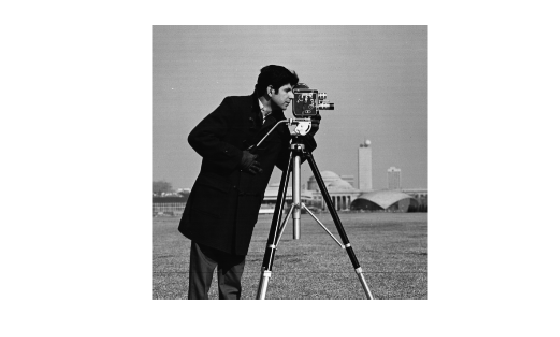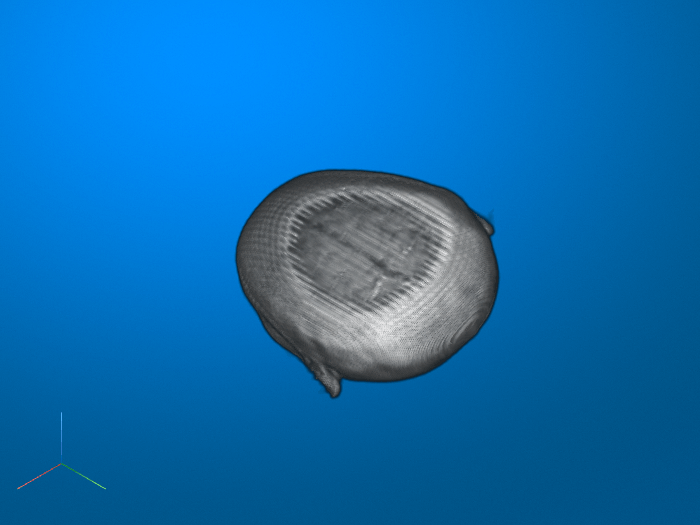Documentation

# imwarp

Apply geometric transformation to image

## Syntax

``B = imwarp(A,tform)``
``B = imwarp(A,D)``
``````[B,RB] = imwarp(A,RA,tform)``````
``B = imwarp(___,interp)``
``````[B,RB] = imwarp(___,Name,Value)``````

## Description

example

````B = imwarp(A,tform)` transforms image `A` according to the geometric transformation `tform`. The input image `A` can be a numeric, logical, or categorical array. `tform` is a geometric transform object. The function returns the transformed image in `B` which is the same class as the input image.```
````B = imwarp(A,D)` transforms image `A` according to the displacement field `D`. ```
``````[B,RB] = imwarp(A,RA,tform)``` transforms a spatially referenced image, specified by the image data `A` and the associated spatial referencing object `RA`. The output is a spatially referenced image specified by the image data `B` and the associated spatial referencing object `RB`.```
````B = imwarp(___,interp)` specifies the type of interpolation to use.```
``````[B,RB] = imwarp(___,Name,Value)``` specifies name-value pair arguments to control various aspects of the geometric transformation.```

## Examples

collapse all

Read grayscale image into workspace and display it.

```I = imread('cameraman.tif'); imshow(I)```Create a 2-D geometric transformation object.

`tform = affine2d([1 0 0; .5 1 0; 0 0 1])`
```tform = affine2d with properties: Dimensionality: 2 T: [3x3 double] ```

Apply the transformation to the image.

```J = imwarp(I,tform); figure imshow(J)```Read 3-D MRI data into the workspace and visualize it.

```s = load('mri'); mriVolume = squeeze(s.D); sizeIn = size(mriVolume); hFigOriginal = figure; hAxOriginal = axes; slice(double(mriVolume),sizeIn(2)/2,sizeIn(1)/2,sizeIn(3)/2); grid on, shading interp, colormap gray```Create a 3-D geometric transformation object. First create a transformation matrix that rotates the image around the y-axis. Then create an `affine3d` object from the transformation matrix.

```theta = pi/8; t = [cos(theta) 0 -sin(theta) 0 0 1 0 0 sin(theta) 0 cos(theta) 0 0 0 0 1]; tform = affine3d(t)```
```tform = affine3d with properties: Dimensionality: 3 T: [4x4 double] ```

Apply the transformation to the image.

`mriVolumeRotated = imwarp(mriVolume,tform);`

Visualize three slice planes through the center of the transformed volumes.

```sizeOut = size(mriVolumeRotated); hFigRotated = figure; hAxRotated = axes; slice(double(mriVolumeRotated),sizeOut(2)/2,sizeOut(1)/2,sizeOut(3)/2) grid on, shading interp, colormap gray```Link the views of both axes together.

`linkprop([hAxOriginal,hAxRotated],'View');`Set the view to see the effect of rotation.

`set(hAxRotated,'View',[-3.5 20.0])`## Input Arguments

collapse all

Image to be transformed, specified as a numeric, logical, or categorical array of any dimensionality.

Data Types: `single` | `double` | `int8` | `int16` | `int32` | `int64` | `uint8` | `uint16` | `uint32` | `uint64` | `logical` | `categorical`

Geometric transformation to perform, specified as an `affine2d`, `projective2d` or `affine3d` object.

• If `tform` is 2-D and `A` has more than two dimensions, such as for an RGB image, then `imwarp` applies the same 2-D transformation to all 2-D planes along the higher dimensions.

• If `tform` is 3-D, then `A` must be a 3-D image volume.

Displacement field, specified as numeric array. The displacement field defines the grid size and location of the output image. Displacement values are in units of pixels. `imwarp` assumes that `D` is referenced to the default intrinsic coordinate system. To estimate the displacement field, use `imregdemons`.

• If `A` is a 2-D grayscale image of size m-by-n, then `D` is m-by-n-by-2. The first plane of the displacement field, `D(:,:,1)`, describes the x-component of additive displacement. `imwarp` adds these values to column and row locations in `D` to produce remapped locations in `A`. Similarly, the second plane of the displacement field, `D(:,:,2)`, describes the y-component of additive displacement values.

• If `A` is a 2-D RGB or 3-D grayscale image of size m-by-n-by-p, then `D` is:

• m-by-n-by-p-by-3. `D(:,:,:,1)` contains displacements along the x-axis, `D(:,:,:,2)` contains displacements along the y-axis, and `D(:,:,:,3)` contains displacements along the z-axis

• m-by-n-by-2, then `imwarp` applies the displacement field to one plane at a time.

Data Types: `single` | `double` | `int8` | `int16` | `int32` | `int64` | `uint8` | `uint16` | `uint32` | `uint64`

Spatial referencing information of the image to be transformed, specified as an `imref2d` object for a 2-D transformation or an `imref3d` object for a 3-D transformation.

Type of interpolation used, specified as one of these values.

Interpolation MethodDescription
`'linear'`Linear interpolation. This is the default interpolation method for numeric and logical arrays.
`'nearest'`Nearest-neighbor interpolation—the output pixel is assigned the value of the pixel that the point falls within. No other pixels are considered. This is the only interpolation method and the default for categorical arrays.
`'cubic'`Cubic interpolation

Data Types: `char` | `string`

### Name-Value Pair Arguments

Specify optional comma-separated pairs of `Name,Value` arguments. `Name` is the argument name and `Value` is the corresponding value. `Name` must appear inside quotes. You can specify several name and value pair arguments in any order as `Name1,Value1,...,NameN,ValueN`.

Example: `J = imwarp(I,tform,'FillValues',255)` uses white pixels as fill values.

Size and location of output image in world coordinate system, specified as the comma-separated pair consisting of `'OutputView'` and an `imref2d` or `imref3d` spatial referencing object. The `ImageSize`, `XWorldLimits`, and `YWorldLimits` properties of the spatial referencing object define the size of the output image and the location of the output image in the world coordinate system. The use of `'OutputView'` is not available when applying displacement fields.

Fill value used for output pixels outside the input image boundaries, specified as the comma-separated pair consisting of `'FillValues'` and a numeric scalar or array. Fill values are used for output pixels when the corresponding inverse transformed location in the input image is completely outside the input image boundaries.

• If the input image is 2-D and the transform is 2-D, the fill value must be scalar.

• If the input image is 3-D and the transform is 3-D, the fill value must be scalar.

• If the input image is N-D and the transform is 2-D, the fill value can be a scalar or an array whose size matches upper dimensions of the input image (3rd to the Nth dimension). For example, if `A` is an RGB image 200-by-200-by-3, the fill value can be a scalar or a 3-by-1 array. To specify a black fill value you can specify `0` or `[0;0;0]`. For another example, if `A` is 4-D with size 200-by-200-by-3-by-10, the fill value can be scalar or a 3-by-10 array.

• If the input image is a categorical array, use the MATLAB `missing` function to specify the fill value. For categorical data, `missing` equates to `<undefined>`.

Example: `128` specifies a middle-gray fill value for any image of data type `uint8`

Example: `0.5` specifies a middle-gray fill value for any image of data type `double`

Example: `[255 255 0]` specifies a yellow fill color for a 2-D RGB image of data type `uint8`

Example: `[1 1 0]` specifies a yellow fill color for a 2-D RGB image of data type `double`

Example: `[0 1 0;1 1 0]'` specifies a green and yellow fill color, respectively, for a series of two RGB images of data type `double`

Pad image to create smooth edges, specified as the logical value `true` or `false`. When set to `true`, `imwarp` pads the input image (with values specified by `FillValues`) to create a smoother edge in the output image. When set to `false`, `imwarp` does not pad the image. Choosing `false` (not padding) the input image can result in a sharper edge in the output image. This sharper edge can be useful to minimize seam distortions when registering two images side by side.

## Output Arguments

collapse all

Transformed image, returned as a numeric, logical, or categorical array of the same size and data type as the input image `A`.

Spatial referencing information of the transformed image, returned as an `imref2d` or `imref3d` spatial referencing object.

## Algorithms

`imwarp` determines the value of pixels in the output image by mapping locations in the output image to the corresponding locations in the input image (inverse mapping). `imwarp` interpolates within the input image to compute the output pixel value.

The following figure illustrates a translation transformation. By convention, the axes in input space are labeled u and v and the axes in output space are labeled x and y. In the figure, note how `imwarp` modifies the spatial coordinates that define the locations of pixels in the input image. The pixel at (1,1) is now positioned at (41,41). In the checkerboard image, each black, white, and gray square is 10 pixels high and 10 pixels wide. For more information about the distinction between spatial coordinates and pixel coordinates, see Image Coordinate Systems.

Input Image Translated